## Wednesday, July 20, 2016

### Showing a bar plot next to a plotted phylogeny

A phytools user asked me yesterday:

“I'm trying to plot a tree side-by-side with a barplot, however the bars are a bit off from the tip labels, how can I correct this?”

This is a great question, because it is very easy to see how this misalignment results:

``````library(phytools)
tree
``````
``````##
## Phylogenetic tree with 26 tips and 25 internal nodes.
##
## Tip labels:
##  R, E, S, W, X, Q, ...
##
## Rooted; includes branch lengths.
``````
``````x
``````
``````##           A           B           C           D           E           F
## -0.32084765  0.38196606 -0.12100495 -1.83763522 -0.92862392 -3.79335667
##           G           H           I           J           K           L
##  0.72976040  0.08573435 -0.88244919 -2.13005682 -2.75687573 -0.31006456
##           M           N           O           P           Q           R
## -1.52206369  1.36749732 -1.61131289  0.21897916 -1.44425879 -0.66191387
##           S           T           U           V           W           X
## -1.07884603 -0.99994662  0.49265512 -0.51313930 -0.82487486 -0.69957983
##           Y           Z
## -3.32350668 -3.86923149
``````
``````par(mfrow=c(1,2))
plotTree(cw<-reorder(tree),mar=c(5.1,2.1,2.1,0.1))
par(mar=c(5.1,0.1,2.1,2.1))
barplot(x[cw\$tip.label],horiz=TRUE,names.arg="")
title(xlab="log(body size)")
``````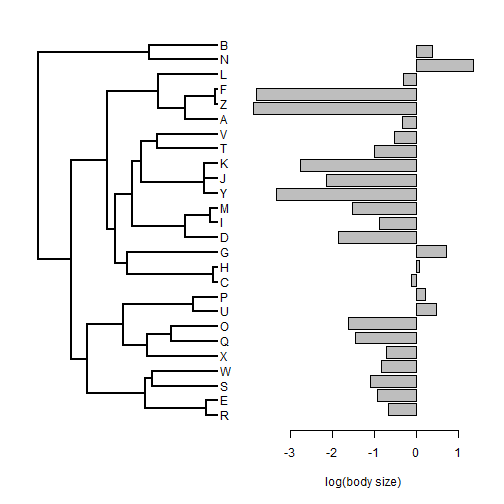At first, this seems OK - but if we add some grid lines we can see that it is not quite….

``````par(mfrow=c(1,2))
plotTree(cw<-reorder(tree),mar=c(5.1,2.1,2.1,0.1))
nulo<-sapply(1:Ntip(cw),function(x) abline(h=x,col="grey"))
par(mar=c(5.1,0.1,2.1,2.1))
tmp<-barplot(x[cw\$tip.label],horiz=TRUE,names.arg="")
title(xlab="log(body size)")
nulo<-sapply(tmp,function(x) abline(h=x,col="grey"))
``````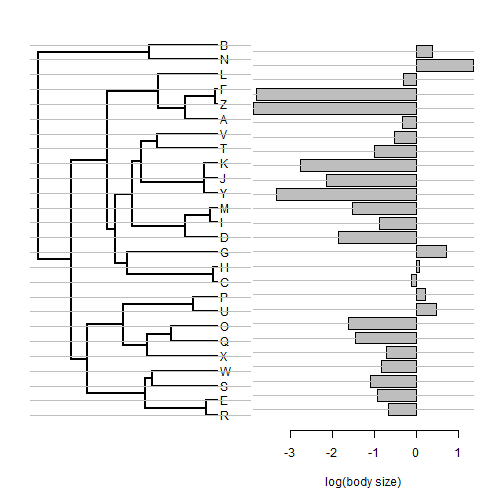The trick is to use the heights of the bars on the barplot to space our tip labels as follows:

``````cw<-reorder(tree)
tmp<-barplot(x[cw\$tip.label],plot=FALSE)
par(mfrow=c(1,2))
plotTree(cw,tips=tmp,mar=c(5.1,1.1,2.1,0.1))
par(mar=c(5.1,0.1,2.1,1.1))
barplot(x[cw\$tip.label],horiz=TRUE,axes=FALSE,
ylim=range(tmp),names.arg="",xlim=range(x))
axis(1)
title(xlab="log(body size)")
``````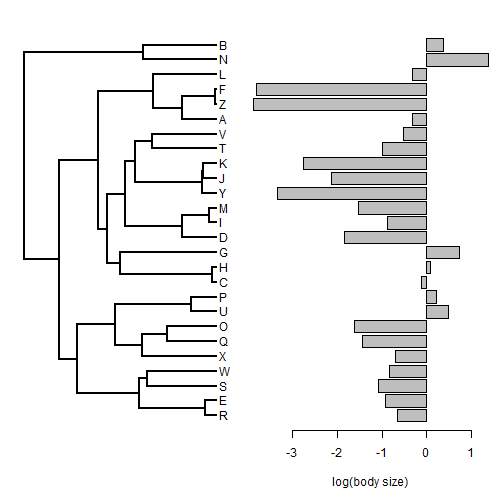and here with our grid lines:

``````cw<-reorder(tree)
tmp<-barplot(x[cw\$tip.label],plot=FALSE)
par(mfrow=c(1,2))
plotTree(cw,tips=tmp,mar=c(5.1,1.1,2.1,0.1))
nulo<-sapply(tmp,function(x) abline(h=x,col="grey"))
par(mar=c(5.1,0.1,2.1,1.1))
barplot(x[cw\$tip.label],horiz=TRUE,axes=FALSE,
ylim=range(tmp),names.arg="",xlim=range(x))
axis(1)
title(xlab="log(body size)")
nulo<-sapply(tmp,function(x) abline(h=x,col="grey"))
``````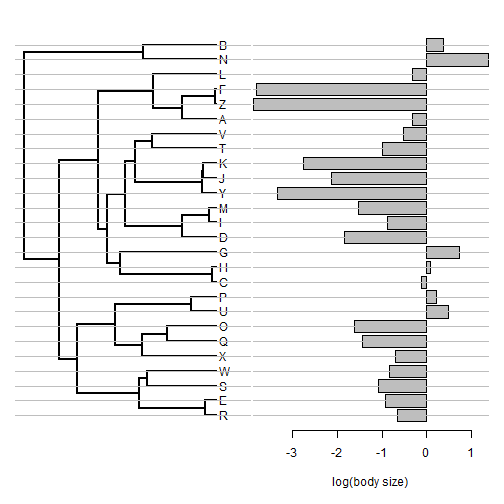Cool. Here it is applied to real data:

``````eel.tree<-read.tree(file=
"http://www.phytools.org/SanJuan2016/data/elopomorph.tre")
"http://www.phytools.org/SanJuan2016/data/elopomorph.csv",
bsize<-setNames(eel.data[,2],rownames(eel.data))
cw<-reorder(eel.tree)
tmp<-barplot(bsize[cw\$tip.label],plot=FALSE,space=0.7)
par(mfrow=c(1,2))
plotTree(cw,tips=tmp,mar=c(5.1,1.1,2.1,0.1),fsize=0.7,
ftype="i",lwd=1)
par(mar=c(5.1,0.1,2.1,1.1))
barplot(bsize[cw\$tip.label],horiz=TRUE,axes=FALSE,
ylim=range(tmp),names.arg="",space=0.7)
axis(1)
title(xlab="body size (cm)")
``````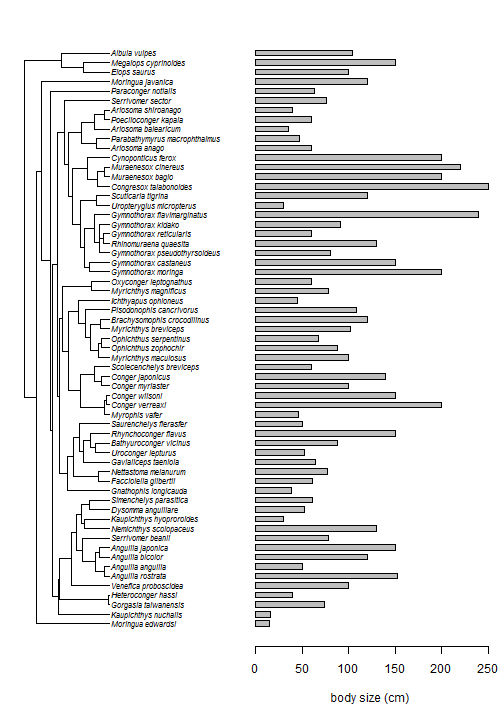That's it.

#### 1 comment:

1.How do we know the length of the tree? how to put the scale?

Note: due to the very large amount of spam, all comments are now automatically submitted for moderation.